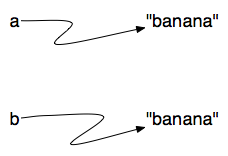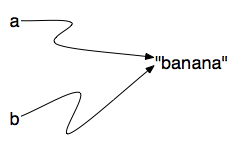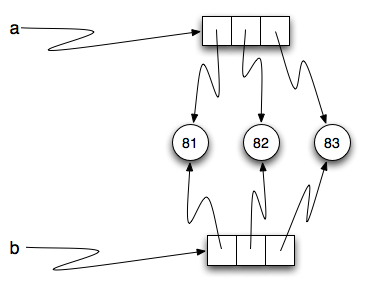# 10.10. Objects and References¶

If we execute these assignment statements,

a = "banana"
b = "banana"


we know that a and b will refer to a string with the letters "banana". But we don’t know yet whether they point to the same string.

There are two possible ways the Python interpreter could arrange its internal states:orIn one case, a and b refer to two different string objects that have the same value. In the second case, they refer to the same object. Remember that an object is something a variable can refer to.

We already know that objects can be identified using their unique identifier. We can also test whether two names refer to the same object using the is operator. The is operator will return true if the two references are to the same object. In other words, the references are the same. Try our example from above.

The answer is True. This tells us that both a and b refer to the same object, and that it is the second of the two reference diagrams that describes the relationship. Since strings are immutable, Python can optimize resources by making two names that refer to the same string literal value refer to the same object.

This is not the case with lists. Consider the following example. Here, a and b refer to two different lists, each of which happens to have the same element values.

The reference diagram for this example looks like this:a and b have the same value but do not refer to the same object.

There is one other important thing to notice about this reference diagram. The variable a is a reference to a collection of references. Those references actually refer to the integer values in the list. In other words, a list is a collection of references to objects. Interestingly, even though a and b are two different lists (two different collections of references), the integer object 81 is shared by both. Like strings, integers are also immutable so Python optimizes and lets everyone share the same object for some commonly used small integers.

Here is the example in codelens. Pay particular attention to the id values.

Activity: CodeLens 10.10.3 (chp09_istrace)